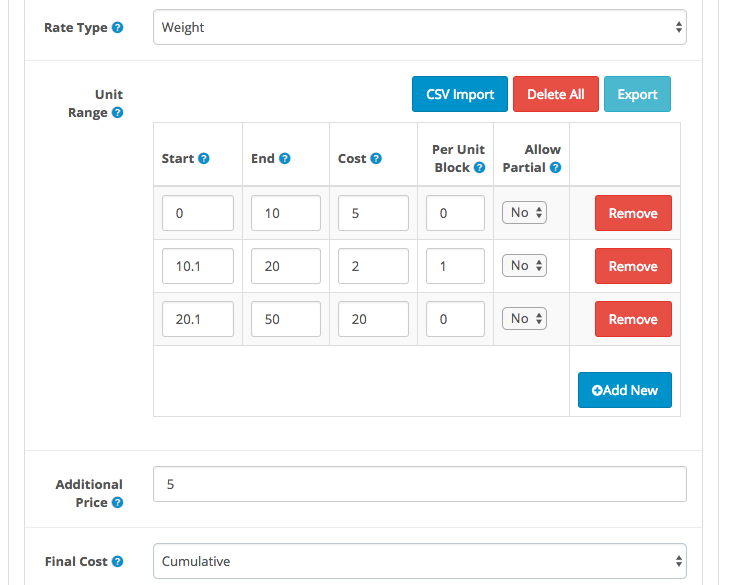# How does Cumulative Price work in XShippingpro?

If you set final cost to Cumulative,  xshippingpro will sup up cost of all unit rows and even applicable Additional Price. Let look into following setting:Before continue into further lets discuss two things.

How doesXshippingpro calculate Per Unit Cost?
If you noticed, I have entered Per Unit Block is 1 for the second unit row.  Since cost is defined \$2 to that row. So each block cost would be \$2. Lets calculate how may blocks out there.
Start: 10.1
End: 20
So Total blocks:  (End – Start) / Per Unit Block = (20 -10.1) / 1 = 9.9 i.e Round value: 10
( One thing is worth to inform that Xshippingpro would not round if you would have set Allow Partial is Yes )
Therefore, Total Cost: 10  x \$2 = \$20
How does Xshippingpro calculate Additional Price?
If xshippingpro find a match among the unit ranges, it will not move forward to Additional Price. But if there no proper matching is found, xshippingpro will move ahead to Additional Price rule and try to calculate shipping cost depending on its value.
By default, xshippingpro calculate additional cost per 1 Unit e.g 1 KG in this case (or whatever Rate Type you defined. If you define Rate type is Quantity, then Per 1 Item) basis unless you define Per Unit Block in the last unit row.
For example, there is no Per Unit Block  defined to the last unit range row. So additional price will be calculated for per 1 KG since Rate type is Weight. Lets say Additional Price is \$10, that means cost for every KG is \$10
If there was Per Unit block defined to the last unit range row, it would calculate additional price per Per Unit block basis. For example, if it was defined 2, additional price would calculate per 2 KG.  Lets say Additional Price is \$10, that means cost for every 2KG is \$10

Let’s proceed to main discussion. Lets say we have cart weight 60KG so what would the final shipping cost according to above setting?

1.  First row:  \$5
2.  Second row:  \$20      (No of blocks: 10 and Cost per block: \$2 ,Therefore, 10 x \$2 = \$20 )
3.  Third row:  \$20
Yes, we have still some weight 10KG pending to be calculated  even after all unit rows are done. Because our last row’s upper limit is 50KG but our cart weight is 60KG
4. Additional Shipping Cost: \$50   ( Additional Price \$5 and we have 10KG to be calculated per 1 KG basis)
Since Final Price cumulative so lets sum up item 1 to 4: ( \$5 +\$20 + \$20 + \$50) = \$95
Therefore, Final shipping cost for 50KG weight would be \$95